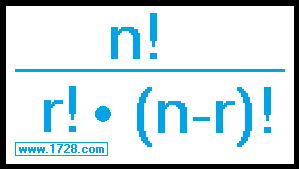* * * * * * * * * * * * * * * * * * * * * * * * * * * * Combination Calculator * * * * * * * * * * * * * * * * * * * * * * * * * * * * If you are looking for a factorial calculator or a derangement calculator, then click here.Input 'r' >>>>> <<<<< Input 'n'
 This calculates all possible combinations you can make when selecting a set of elements ('r'), from a larger set of elements ('n'). For example, in how many ways can you select three letters from the set of a, b, c, d, e and f? Basically, you are selecting 3 letters from a set of 6. Using the above calculator we see this can be done in 20 ways. The above formula also calculates 20 ways. 6! ÷ (3! • (6 -3)!)   =   720 ÷ (6 • 6)   =   20 The "brute force" method also generates 20 combinations: abc, abd, abe, abf, acd, ace, acf, ade, adf, aef bcd, bce, bcf, bde, bdf, bef, cde, cdf, cef, def

 For another example, let's look at the state of New York's Sweet Million lottery game. To play this lottery, you choose 6 numbers from a field of 40. The order in which you pick the numbers does not matter: for example (1, 2, 3, 4, 5, 6) is exactly the same as (3, 5, 1, 6, 2, 4) Repetition is not allowed. In other words, you cannot choose 5, 5, 8, 13, 19, 31 as a lottery number. Given these conditions, in how many ways, can you choose 6 numbers from 40? You could use the formula listed above or use the calculator. Here, you would input 40 for 'n' and 6 for 'r', click calculate and your answer is 3,838,380.

"R E T U R N"